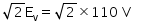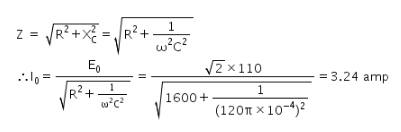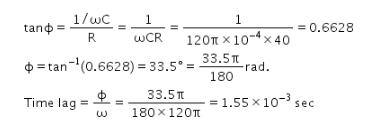# A 100 micro farad capacitor in series with a 40 ohms resistance

A 100 microfarad capacitor in series with a 40 ohms resistance is connected to a 110V , 60 Hz supply (a) what is the maximum current in the circuit (b) what is the time lag between the current maximum and voltage maximum.

Given:
C = 100 μF = 100 × 10-6 F = 10-4 F
R = 40 Ω
Ev = 110 volt
E0 =v = 60 Hz
ω = 2Πv = 120 Π rad/s
I0 = ?
In RC circuit, asIn RC circuit, voltage lags behind the current by phase angle Φ,
where